Flight Testing A forum for test pilots, flight test engineers, observers, telemetry and instrumentation engineers and anybody else involved in the demanding and complex business of testing aeroplanes, helicopters and equipment.

# Machmeter

Join Date: Jun 2012
Location: Vietnam
Posts: 10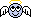Machmeter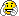Somebody please show me the derivation of the formula that drives the MachmeterJoin Date: Jun 2001
Location: England
Posts: 955
Speed of sound in kts =38.94* sqrt absolute temperaturePer Ardua ad Astraeus

Join Date: Mar 2000
Location: UK
Posts: 18,584
He asked for the 'derivation', not the formula!More bang for your buck

Join Date: Nov 2005
Location: land of the clanger
Age: 76
Posts: 3,511
Scroll through the pages of this book, it may help:

USAF Flight Test Engineering HandbookJoin Date: Jun 2001
Location: England
Posts: 955
Ooops sorry. Looking forward to seeing the derivation.

(Edit - in fact i just googled it. Not sure I'm any more 'complete' for knowing it.) Why do you want to know it?

Last edited by 18greens; 1st Jul 2012 at 20:03.Join Date: Jun 2012
Location: Vietnam
Posts: 10
Machmeter

Well....Pilots have to know the principle and working method of their flight instruments as I'm sure you are aware. The Machmeter is just one of them.Per Ardua ad Astraeus

Join Date: Mar 2000
Location: UK
Posts: 18,584
Can we clarify here? Are you simply asking how it works, or do you really want the 'derivation' of the equations ie how the dimensions of the various factors are determined? EG why sq rt Abs T? Why 39.84? If so, as 18g says - why?Join Date: Jun 2012
Location: Vietnam
Posts: 10
Machmeter formula

Wow sir. I think I'm getting connection! Yes the basic instrument relies on inputs of Static pressure and Dynamic pressure. There is a formula which relates these pressures to the Mach number which we know is the TAS/local Speed of Sound and is used to run (program) the instrument.
I think the derivation starts from the velocity of sound being proportional to the sq. rt. of the temperature, involves the gas equation ( I think the PV=mRT one) and gives a quite simple equation for Mach number ( I know latest designs include air data computer input but that is a much later sophistication). I'm looking for the several equations which when manipulated and simplified give the relation ship.
You really sound like you can show me!Join Date: Mar 2008
Location: London
Posts: 76
A simple approach

My log in does not support clever symbols. Any treatise will do but pp65-88 of Philip G Hill & R Peterson ISBN 0-201-14569-2, Dewey classification 629.1328 published by Addison Wesley does the job.

Caveats are:- the assumption that flow can be assumed as one-dimensional through the control volume is tosh; but on this basis pressure, shear and body forces satisfy a simple momentum equation provided that the fluid is a perfect gas and gravitational potential energy can be neglected; therefore stagnation enthalpy contains only two terms; therefore stagnation temperature is a function solely of cp, dT and therefore the ratio of specific heats; for an infinitesimally thin slice of control volume it is customary to neglect shear force [but what about body forces?] so that for an isentropic flow -dp = rho a du; thus du can be eliminated by simultaneous equations and a*a = kRT.

That is as far as Hill and Peterson go in the quoted first four pages leaving the difficult stuff to the rest of the chapter, sadly glossing over choking flow but non-isentropic flow and shocks are handled.

Boundary layer and the limitation of simple control volume theory are expounded in Chapter Four.

Last edited by mathy; 5th Jul 2012 at 15:32. Reason: typingJoin Date: Dec 2006
Location: The No Trangression Zone
Posts: 2,049
Mathy...that's more of a description of the flow more than what the Mach mter is using, as an aside, Old Smokey has posted the
EXACT and CORRECT equation like a million times here...READ!! he revealed important information on the topic of Mach meter calibrationThe derivation would be as follows:

Bernoulli's equation derived ultimately in terms of the thermodynamic equations is obtained from the equation written in terms sound velocity 'a'...the slope of the isoentrope passing through -rho^2*a^2 and that is equivalent to [dp/dv]s ...

so a =[1/rho^2* [dp/dv]s}^1/2, since Mach= TAS/a
since TAS is CAS corrected for compressibility(EAS),...in turn EAS correct for altitude density ration 'sigma' is TAS... the (dp/dv) term is determined using the adiabatic gas laws for adiabatic compressibility to introduce a correction term so rewriting all of the terms for Mach number CAS (dp/dv)*sigma/[1/rho^2* [dp/dv]s]^1/2) after tons of algebra that I'm not doing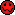...will give you the Mach meter equation... there are other circumscribing elementary equation from thermodynamics that need to be applied and the above equation are in turn derived from basic principles of mechanics and thermodynamics...from fundamental thermodynamics laws the actual equation I'd estimate at 10 pages of algebra and calculus, maybe much more, if you where to really attempt the derivation from basic principles, I don't know, ...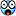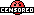I assume the original poster has some finite background in differential equations to be asking that type of question...I'm not gonna duit...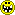I forgot to add the slope of where the velocity gradient 'dv' approaches zero, or the stagnation point, or in yet other words,...lim dv--->0 ...but can not equal zero...as then the whole set of functions would be undefined...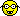I think... I got this right, please double check, and let me no if I made an error I'm not looking at any txt book right now, not available...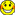Last edited by Pugilistic Animus; 8th Jul 2012 at 16:29. Reason: a little math error...and some clarificationsJoin Date: Dec 2006
Location: The No Trangression Zone
Posts: 2,049
here's a link to an earlier post I made about adiabatic gas laws...if it helps...http://www.pprune.org/flight-testing...-question.html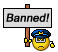Join Date: Apr 2009
Location: down south
Age: 71
Posts: 13,229
Gentlemen,

The Machmeter has no inputs of TAS or LSS. To do so it would need to be a computer.

The Machmeter is a pressure instrument which is basically an ASI with an altimeter capsule added.

It measures the ratio of dynamic to static pressure.Join Date: Dec 2006
Location: The No Trangression Zone
Posts: 2,049
The Machmeter has no inputs of TAS or LSS. To do so it would need to be a computer.

The Machmeter is a pressure instrument which is basically an ASI with an altimeter capsule added.

It measures the ratio of dynamic to static pressure.
yes that is true the inputs are Ps-q but q for compressible flow is a comp-lex function the paramters p and q have to be converted to a mach number the function for mach number can be wirtten entirely in terms of those paramterss Ps and q. in the classical Machmeter [stem gusge] the computations are made by the cam gearing form the bellows....

that being said I made an error in the above very cumbersome functions

I'll rewrite it here:
(Ps -q) =CAS

Ps-q= Ps-([dp/dv]) = ...CAS corrected for compressibilty=EAS or

EAS corrected for altitude density ratio 'sigma' =TAS this is what I forgot above
the speed of sound 'a' written as a pressure function =a =[1/rho^2* [dp/dv]s}^1/2

Ps-([dp/dv])*sigma=TAS or 'V' Mach =V/a

V/a =Ps-([dp/dv])*sigma/[1/rho^2* [dp/dv]s}^1/2=M=SQR (5 X (Qc/Ps + 1)^((Y-1)/Y) - 1) ...this is from Old Smokey... gamma's come from adiabatic gas laws, but it still has to be solved...but the two will eventually lead to one another
he other major error I made was putting in a0...I don't know why I did that...so in the above I incorrectly included the ratio a0/a it does not belong...and I forgot to multiply EAS by the altitude density ratio...I put the corrections above...From Old Smokey
Before I'm accused of over-simplification, take a look at the formulae by which the Airspeed Indicator and the Machmeter are calibrated -

Vc = SQR((Y/(Y-1)) X Po/Qc X ((Qc/Po + 1)^((Y-1)/Y) - 1) X SQR (2 X Qc/Rho0)

M = SQR (5 X (Qc/Ps + 1)^((Y-1)/Y) - 1)

(NOTE - For flight at altitude, substitute Ps for Po in the Vc formula).

Where -

Vc = Calibrated Air Speed, ft/sec
M = Mach Number
Y = A constant, being the ratio of specific heat of air at constant pressure to the specific heat of air at constant volume = 1.4
Po = Sea Level Air Pressure
Ps = Static Pressure
Qc = Impact Pressure
Rho0 = Standard Sea level air density

Before I'm accused of over-simplification, take a look at the formulae by which the Airspeed Indicator and the Machmeter are calibrated -

Vc = SQR((Y/(Y-1)) X Po/Qc X ((Qc/Po + 1)^((Y-1)/Y) - 1) X SQR (2 X Qc/Rho0)

M = SQR (5 X (Qc/Ps + 1)^((Y-1)/Y) - 1)

(NOTE - For flight at altitude, substitute Ps for Po in the Vc formula).

Where -

Vc = Calibrated Air Speed, ft/sec
M = Mach Number
Y = A constant, being the ratio of specific heat of air at constant pressure to the specific heat of air at constant volume = 1.4
Po = Sea Level Air Pressure
Ps = Static Pressure
Qc = Impact Pressure
Rho0 = Standard Sea level air density

Last edited by Pugilistic Animus; 8th Jul 2012 at 16:43.Per Ardua ad Astraeus

Join Date: Mar 2000
Location: UK
Posts: 18,584
There you go, Hurri!Sorted. Good stuff, fellows/fellassiesJoin Date: Jun 2012
Location: Vietnam
Posts: 10
Machmeter

I don't deny that the equations for Vc and M which Pugilistic Animus quotes from Old Smokey are right. Your efforts on the subject are both appreciated.
However in the interest of simplicity which I fancy truly exists here seeing:
M= P/S
where M is Mach Number, P is the Dynamic pressure and S is the Static pressure as Lightning Mate says is what I'd been hoping to see(!).
So putting it to the test; if that observant (who ever is not!) pilot in his beaut old Hunter sees Mach 0.898 on his Machmeter his P is 890 mb and his S is 990 mb (mb: millibars, old units, but dont worry). Yes?
But in a faster ship with his Machmeter indicating 1.3 his Dynamic pressure has to be 1287 mb for a Static pressure of 990mb. Is that a fact? How is that ?
Lightning Mate, you've made sterling pointers so far can you show me how P/S is true, too? ( how it is derived?)Join Date: Apr 2009
Location: down south
Age: 71
Posts: 13,229
Yes - I can do that for you, but I have no access to this site at work, so would you kindly wait until after working hours.Per Ardua ad Astraeus

Join Date: Mar 2000
Location: UK
Posts: 18,584
Hurri -
However in the interest of simplicity which I fancy truly exists here seeing: M= P/S
- cannot see where you get that from? As you can see the Machmeter does not simply " measure the ratio of dynamic to static pressure" in real terms..

In post #13 you were given

M = SQR (5 X (Qc/Ps + 1)^((Y-1)/Y) - 1) which is where your "P/S" sits.

"P" is 'derived' from "Total pressure"
"S" " " " "Static Pressure"

What we cannot fathom is why the need to go to the very root of this equation? Why do you need to know please? Why does this not satisfy your thirst for knowledge? Have the search engines failed to answer?

What does "can you show me how P/S is true," mean?

I'm looking for the several equations which when manipulated and simplified give the relation ship.
- I would observe you have them!Join Date: Jun 2004
Location: Australia
Posts: 1,844
Pugilistic Animus, BOAC,

Thanks gents for providing the link to some of my old 'work', that's going back a bit now.

Hurricane6, I regret that there's not going to be any simplification of the formula used for Machmeter calibration, the essentials of a complete and correct calculation are there. The only 'relief' that I can provide is the reduction of the (Y-1)/Y exponent to 2/7.

I note that in one of your intermediate posts you refer to supersonic flight. The formulae for Subsonic (including transonic) and Supersonic flight are -

SUBSONIC (Bernoulli's equation for M<1)

M = SQR (5 X (Qc/Ps + 1)^((Y-1)/Y) - 1)

SUPERSONIC (Rayleigh Supersonic Pitot equation)

M= .88128485 SQR ((Qc/Ps +1) X (1-1/(7 X M^2)^2.5)

(For Qc measured behind the normal shock).

(M appears on both sides of the supersonic equation. The most practical solution of the supersonic equation is to make initial Mach calculation from the subsonic formua, and then by several iterations apply successive Mach number results to the supersonic algorithm as differential between successive results approaches zero).Join Date: Jun 2012
Location: Vietnam
Posts: 10
MachmeterJoin Date: Jun 2012
Location: Vietnam
Posts: 10
Machmeter

As soon as you have time Lightning Mate I'll be glad to hear more from you. Many thanksShow Printable VersionEmail this Page